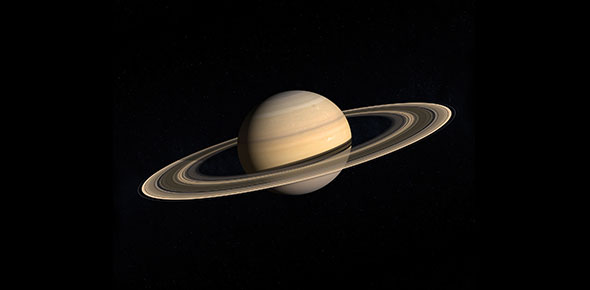# Newton And The Saturn V Rocket

10 Questions | Attempts: 146
ShareSettings• 1.
Who was the scientist who gave us laws of motion?
• A.

Albert Einstein

• B.

Isaac Newton

• C.

James Watt

• D.

Thomas Edison

• 2.
How many laws of motion are there?
• A.

One

• B.

Two

• C.

Three

• D.

Four

• 3.
What is another name for the first law of motion?
• A.

Acceleration

• B.

Force

• C.

Momentum

• D.

Inertia

• 4.
Which law of motion explains why we need to wear seatbelts?
• A.

First Law of Motion

• B.

Second Law of Motion

• C.

Third Law of Motion

• D.

National Highway Traffic and Safety Administration

• 5.
Which law says that force is equal to mass x acceleration?
• A.

Third law of motion

• B.

Second law of motion

• C.

First law of motion

• D.

None of the above

• 6.
Which law says that heavier objects require more force to move or accelerate than lighter objects?
• A.

Law of Conservation of Momentum

• B.

First law of motion

• C.

Second law of motion

• D.

Third law of motion

• 7.
Which law explains how rockets are launched into space?
• A.

Law of Conservation of Momentum

• B.

First law of motion

• C.

Second law of motion

• D.

Third law of motion

• 8.
Which law says that for every action there is an equal and opposite reaction?
• A.

Law of thermodynamics

• B.

Law of Conservation of Momentum

• C.

Third law of motion

• D.

Second law of motion

• 9.
After they removed their gloves and they were floating in the Apollo capsule, the gloves stopped moving.
• A.

True

• B.

False

• 10.
When they were thrown forward into their seat harnesses, the astronauts were demonstrating the First Law of Motion.
• A.

True

• B.

False

## Related TopicsBack to top
×

Wait!
Here's an interesting quiz for you.